scale_x_discrete() and scale_y_discrete() are used to set the values for discrete x and y scale aesthetics. For simple manipulation of scale labels and limits, you may wish to use labs() and lims() instead.

## Usage

scale_x_discrete(..., expand = waiver(), guide = waiver(), position = "bottom")

scale_y_discrete(..., expand = waiver(), guide = waiver(), position = "left")

## Arguments

...

Arguments passed on to discrete_scale

palette

A palette function that when called with a single integer argument (the number of levels in the scale) returns the values that they should take (e.g., scales::hue_pal()).

breaks

One of:

• NULL for no breaks

• waiver() for the default breaks (the scale limits)

• A character vector of breaks

• A function that takes the limits as input and returns breaks as output. Also accepts rlang lambda function notation.

limits

One of:

• NULL to use the default scale values

• A character vector that defines possible values of the scale and their order

• A function that accepts the existing (automatic) values and returns new ones. Also accepts rlang lambda function notation.

drop

Should unused factor levels be omitted from the scale? The default, TRUE, uses the levels that appear in the data; FALSE uses all the levels in the factor.

na.translate

Unlike continuous scales, discrete scales can easily show missing values, and do so by default. If you want to remove missing values from a discrete scale, specify na.translate = FALSE.

na.value

If na.translate = TRUE, what aesthetic value should the missing values be displayed as? Does not apply to position scales where NA is always placed at the far right.

aesthetics

The names of the aesthetics that this scale works with.

scale_name

The name of the scale that should be used for error messages associated with this scale.

name

The name of the scale. Used as the axis or legend title. If waiver(), the default, the name of the scale is taken from the first mapping used for that aesthetic. If NULL, the legend title will be omitted.

labels

One of:

• NULL for no labels

• waiver() for the default labels computed by the transformation object

• A character vector giving labels (must be same length as breaks)

• An expression vector (must be the same length as breaks). See ?plotmath for details.

• A function that takes the breaks as input and returns labels as output. Also accepts rlang lambda function notation.

super

The super class to use for the constructed scale

expand

For position scales, a vector of range expansion constants used to add some padding around the data to ensure that they are placed some distance away from the axes. Use the convenience function expansion() to generate the values for the expand argument. The defaults are to expand the scale by 5% on each side for continuous variables, and by 0.6 units on each side for discrete variables.

guide

A function used to create a guide or its name. See guides() for more information.

position

For position scales, The position of the axis. left or right for y axes, top or bottom for x axes.

## Details

You can use continuous positions even with a discrete position scale - this allows you (e.g.) to place labels between bars in a bar chart. Continuous positions are numeric values starting at one for the first level, and increasing by one for each level (i.e. the labels are placed at integer positions). This is what allows jittering to work.

Other position scales: scale_x_binned(), scale_x_continuous(), scale_x_date()

## Examples

ggplot(diamonds, aes(cut)) + geom_bar()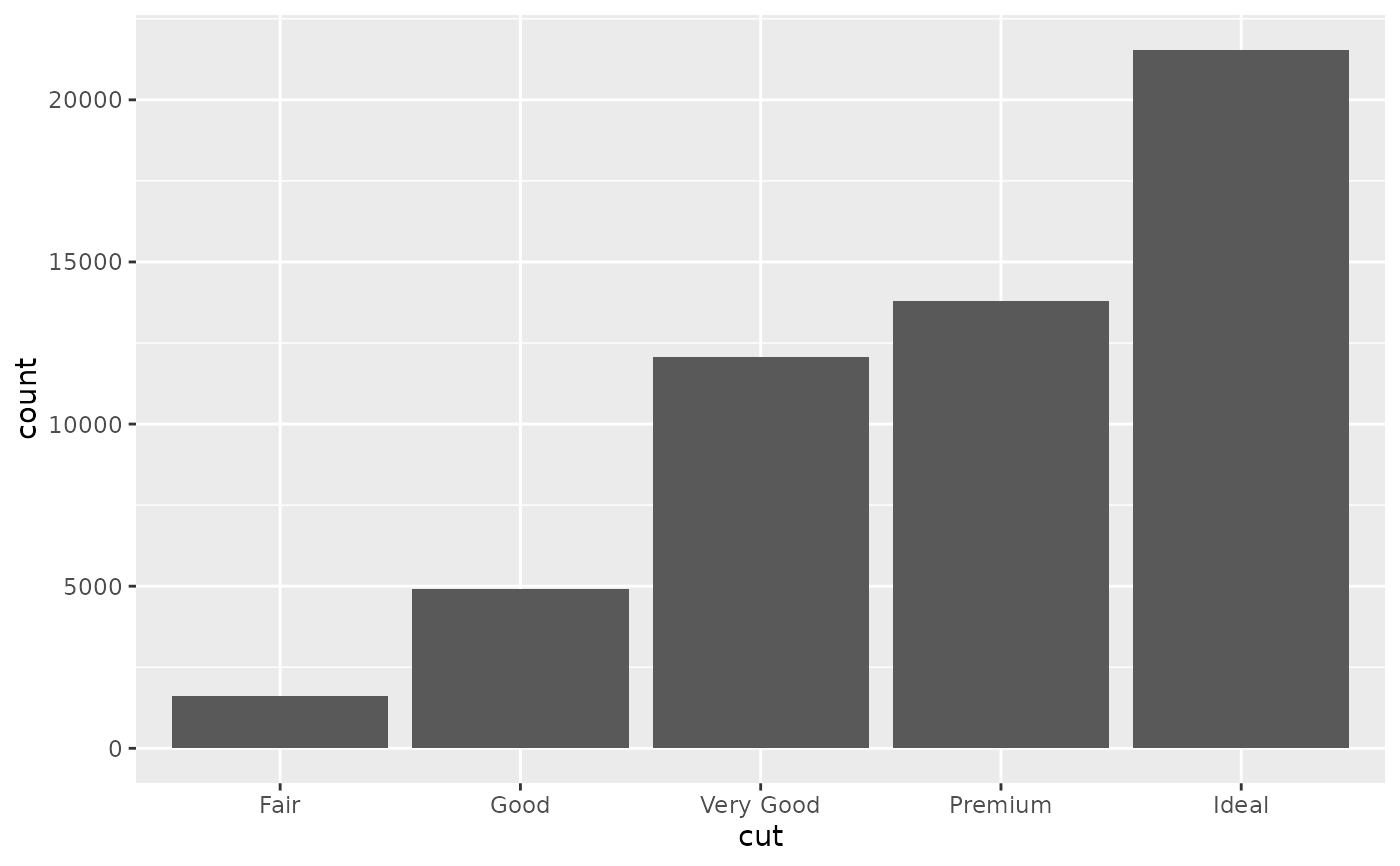# \donttest{
# The discrete position scale is added automatically whenever you
# have a discrete position.

(d <- ggplot(subset(diamonds, carat > 1), aes(cut, clarity)) +
geom_jitter())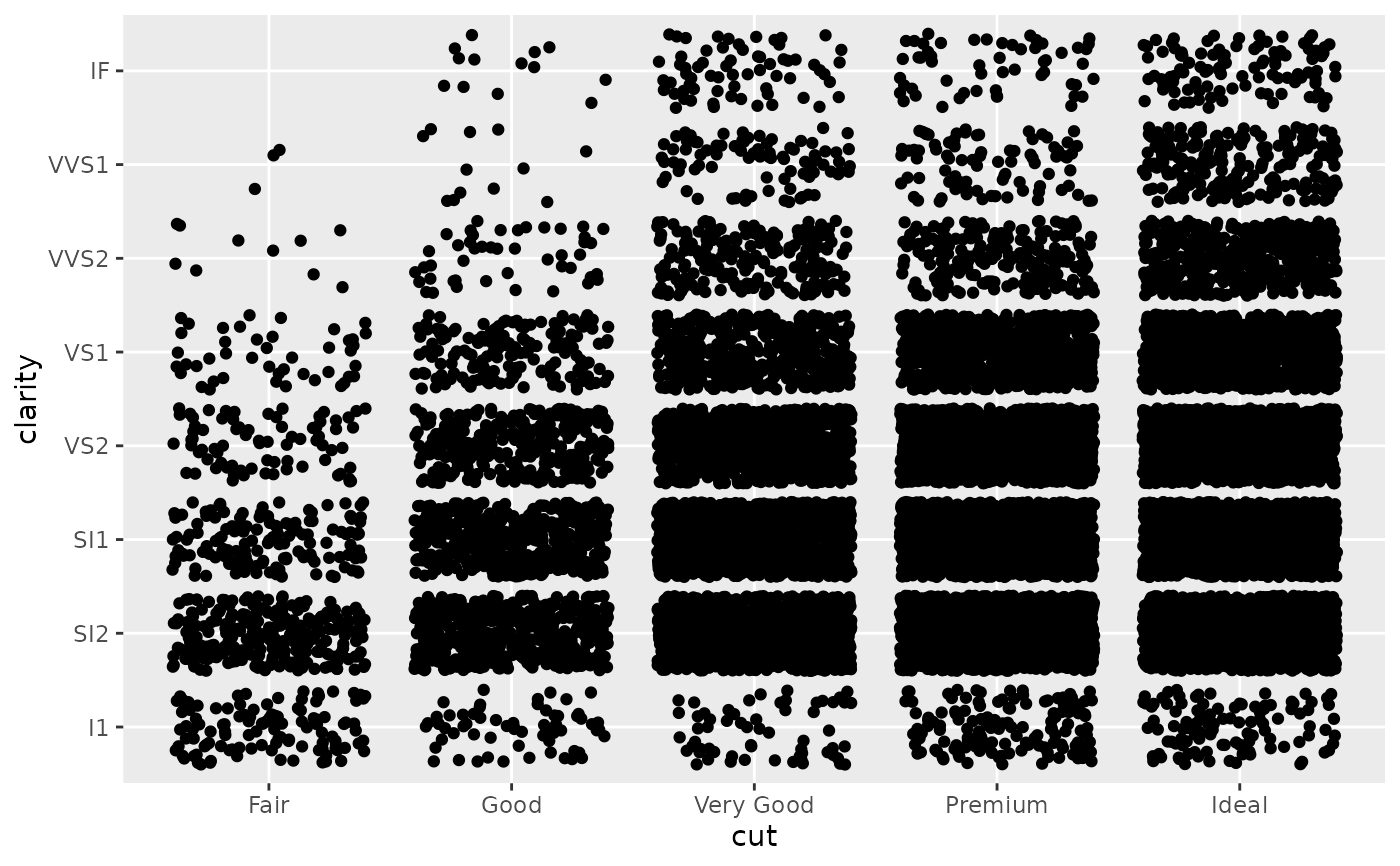d + scale_x_discrete("Cut")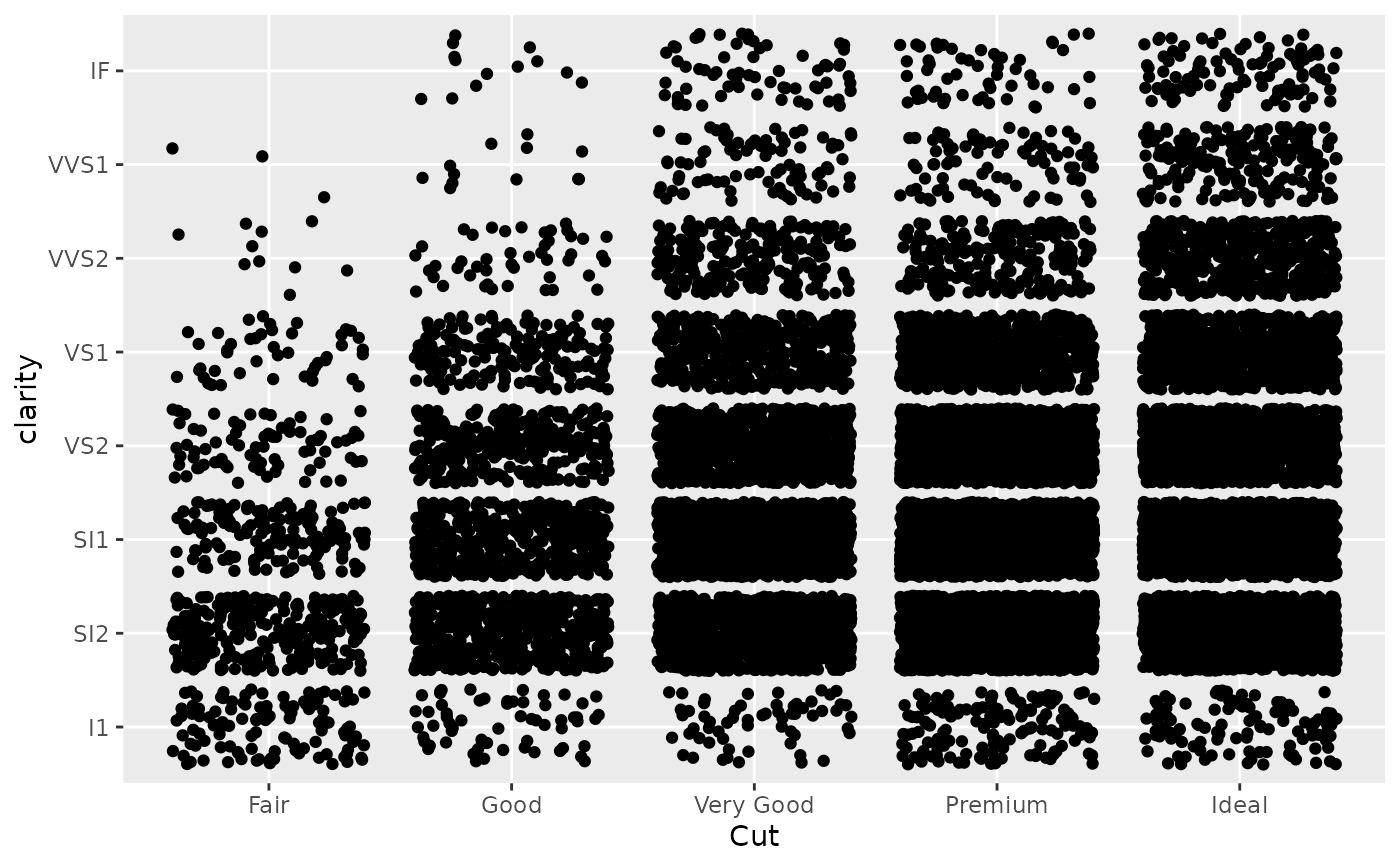d +
scale_x_discrete(
"Cut",
labels = c(
"Fair" = "F",
"Good" = "G",
"Very Good" = "VG",
"Perfect" = "P",
"Ideal" = "I"
)
)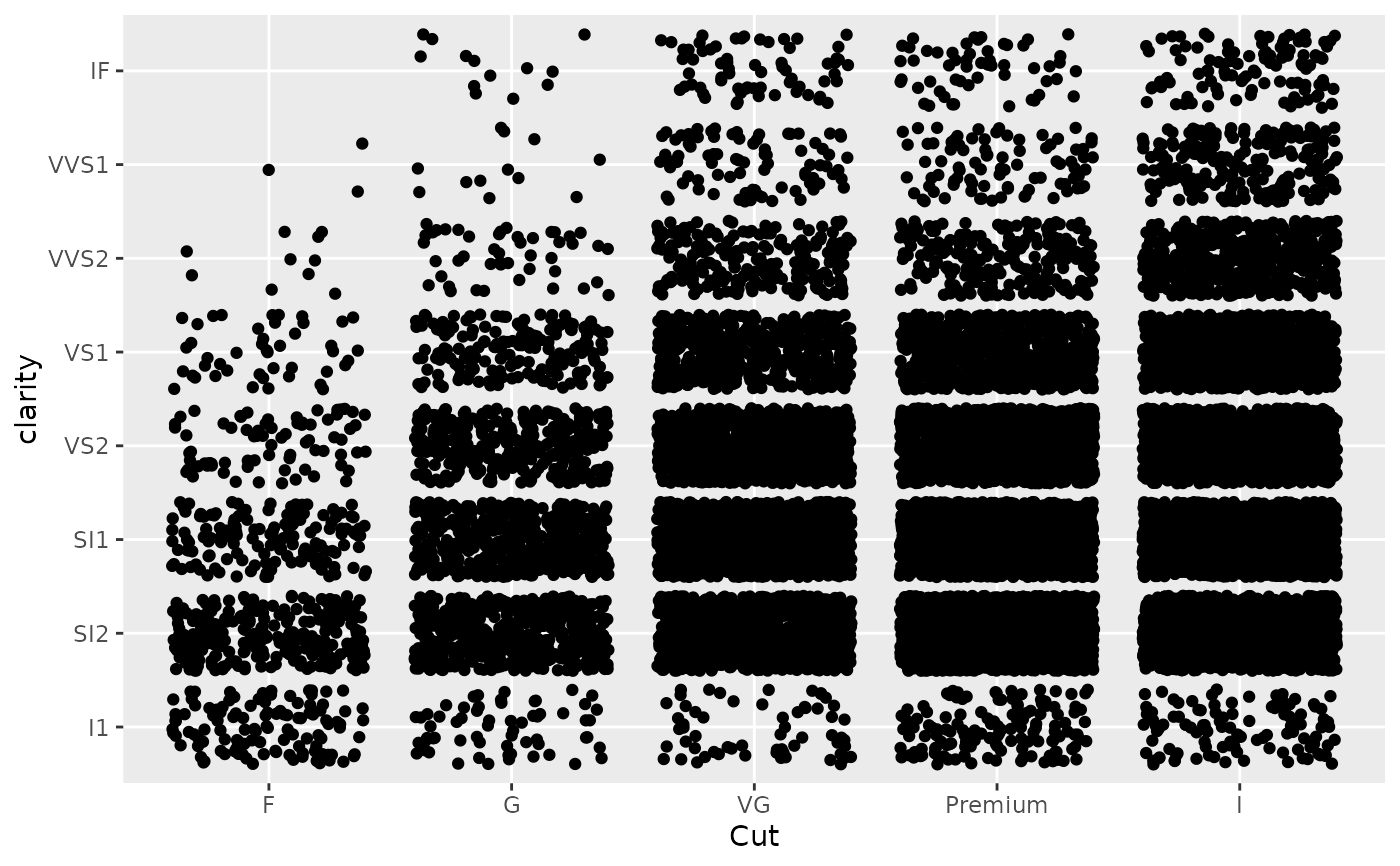# Use limits to adjust the which levels (and in what order)
# are displayed
d + scale_x_discrete(limits = c("Fair","Ideal"))
#> Warning: Removed 11189 rows containing missing values (geom_point()).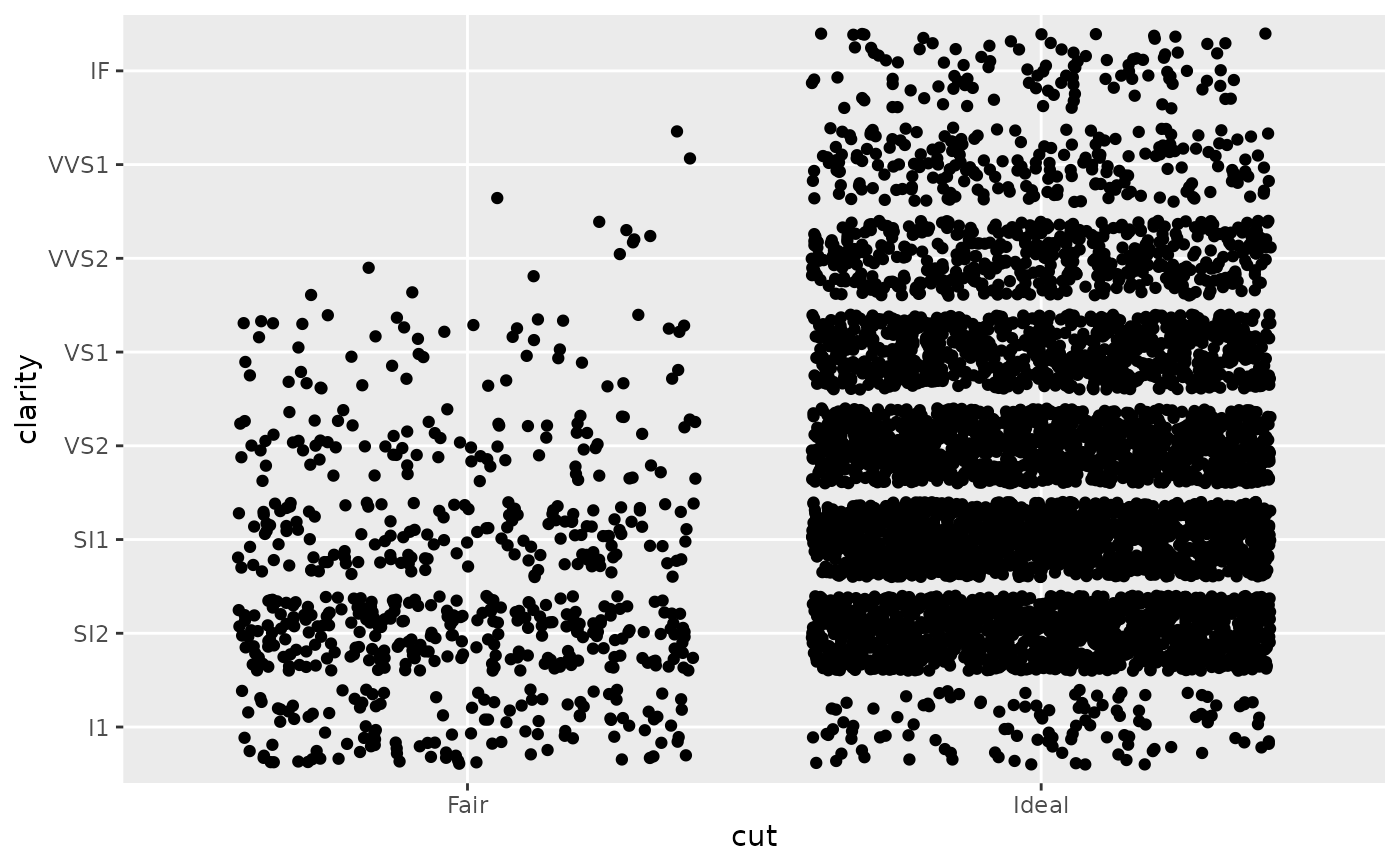# you can also use the short hand functions xlim and ylim
d + xlim("Fair","Ideal", "Good")
#> Warning: Removed 9610 rows containing missing values (geom_point()).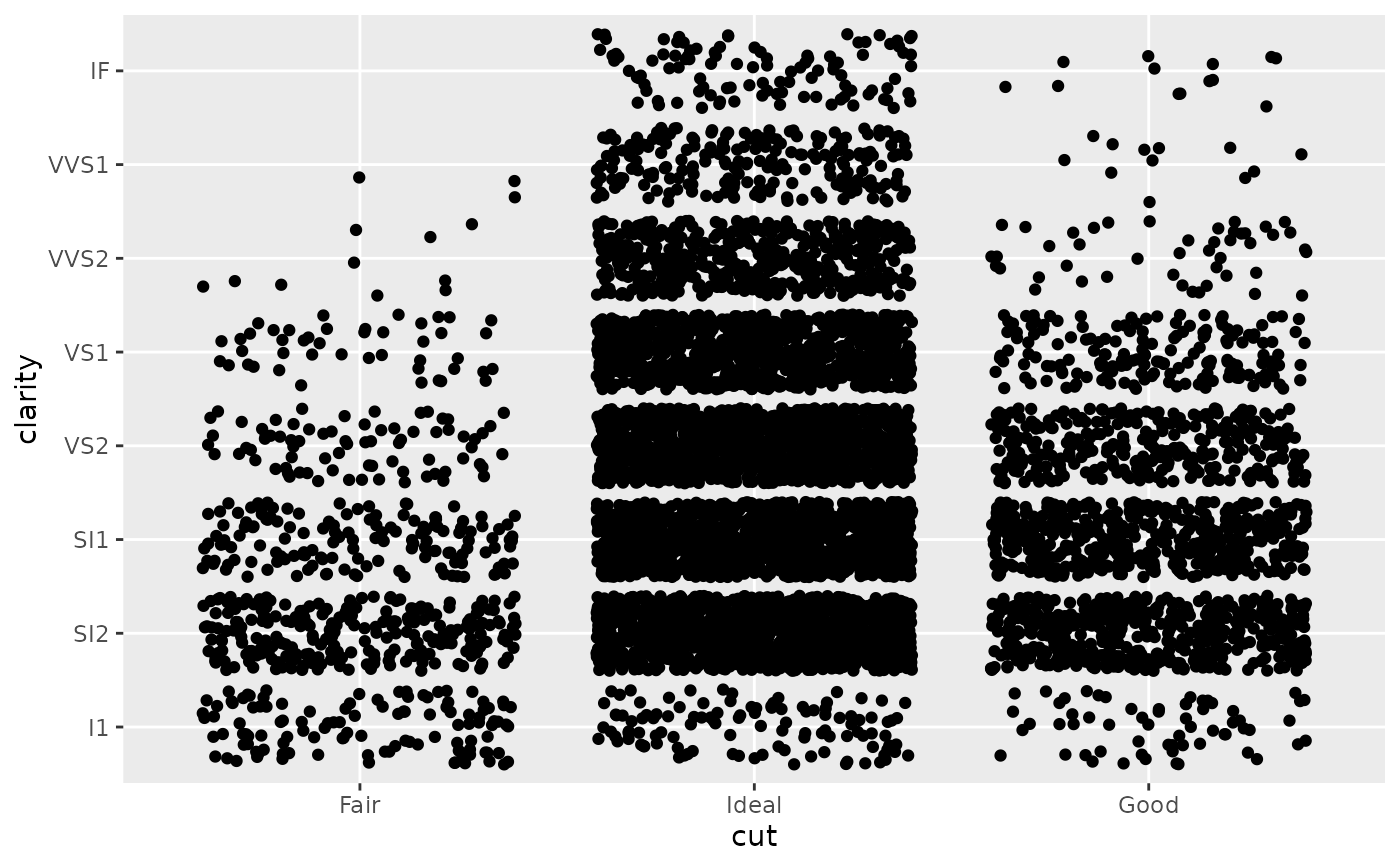d + ylim("I1", "IF")
#> Warning: Removed 16770 rows containing missing values (geom_point()).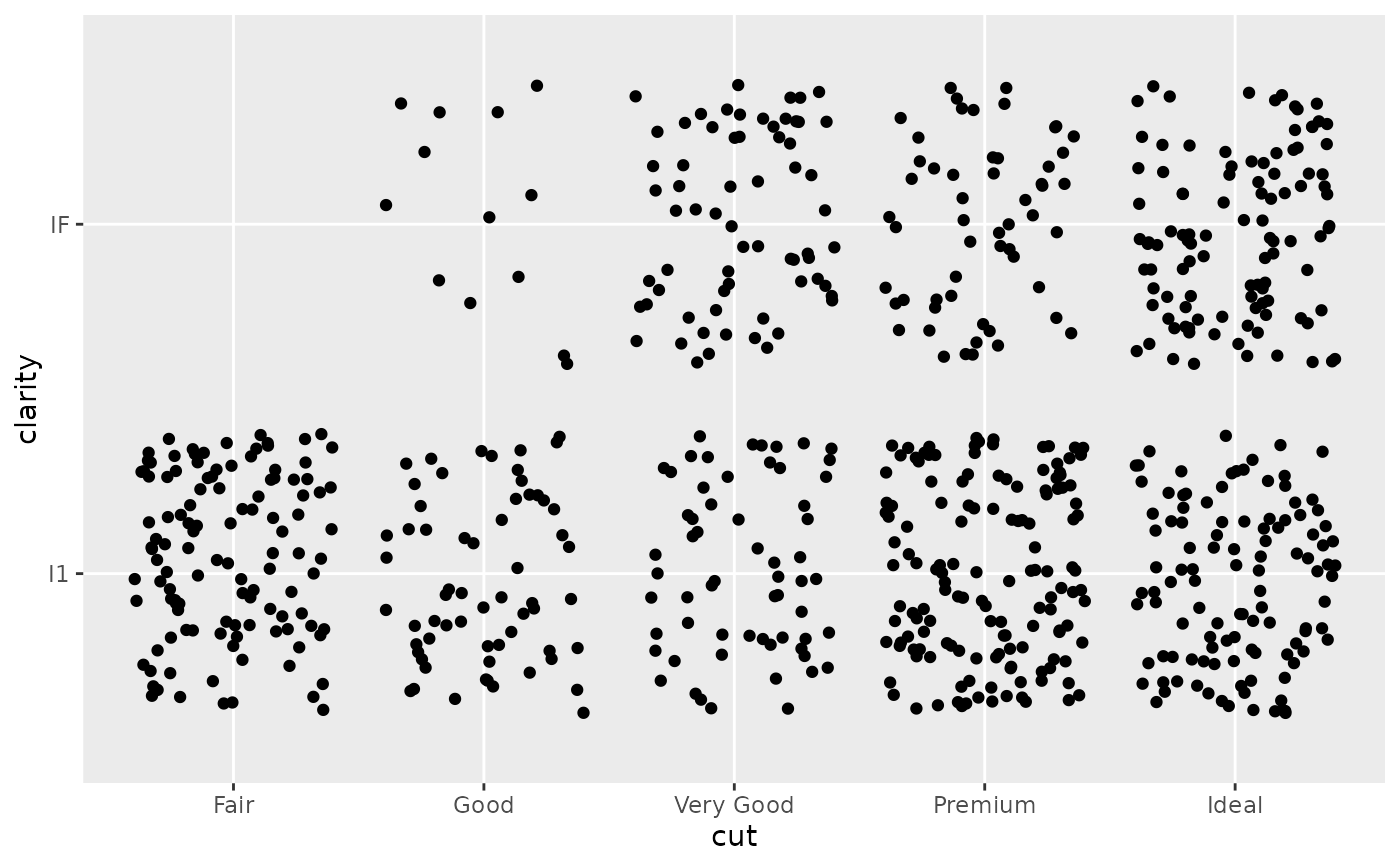# See ?reorder to reorder based on the values of another variable
ggplot(mpg, aes(manufacturer, cty)) +
geom_point()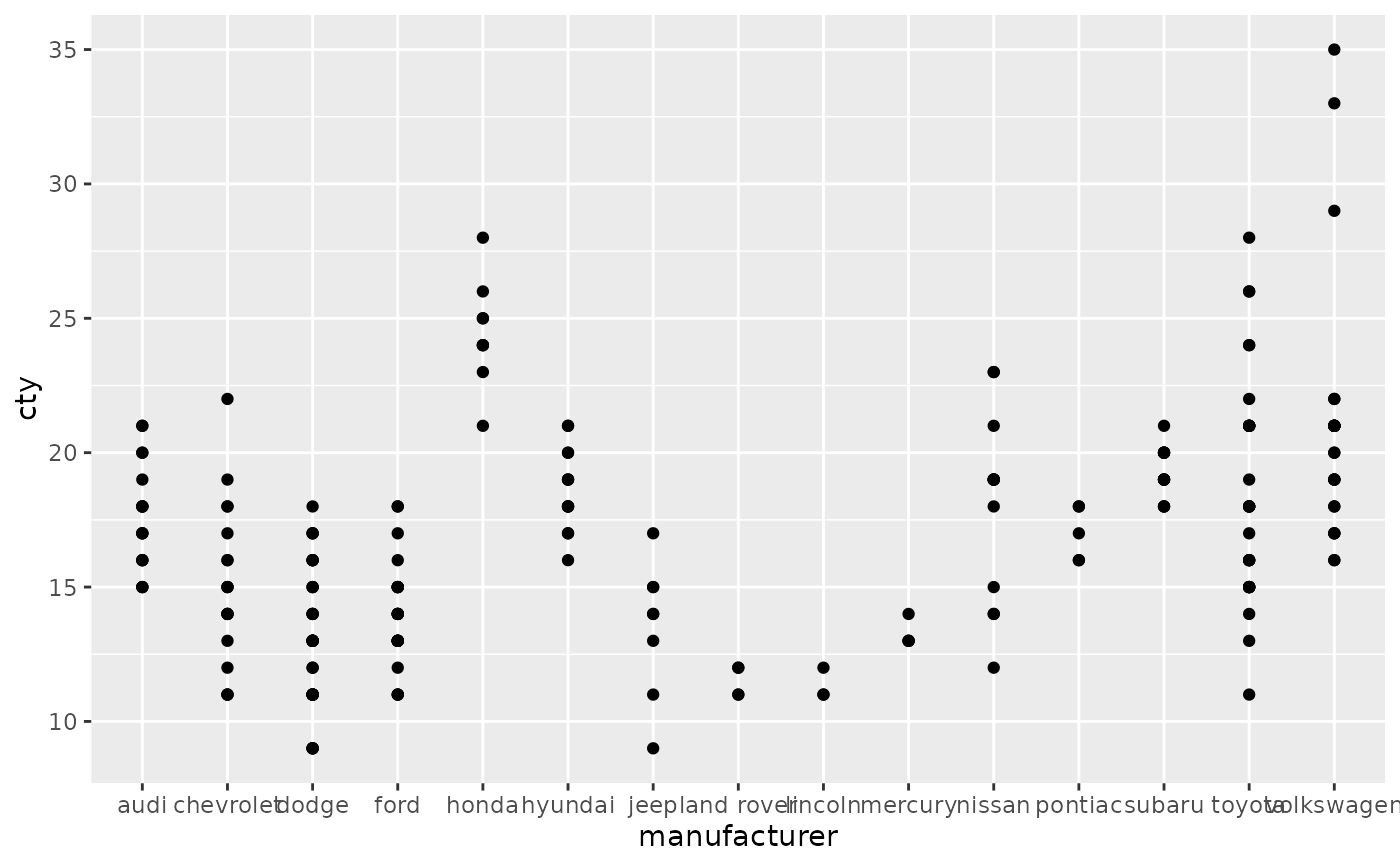ggplot(mpg, aes(reorder(manufacturer, cty), cty)) +
geom_point()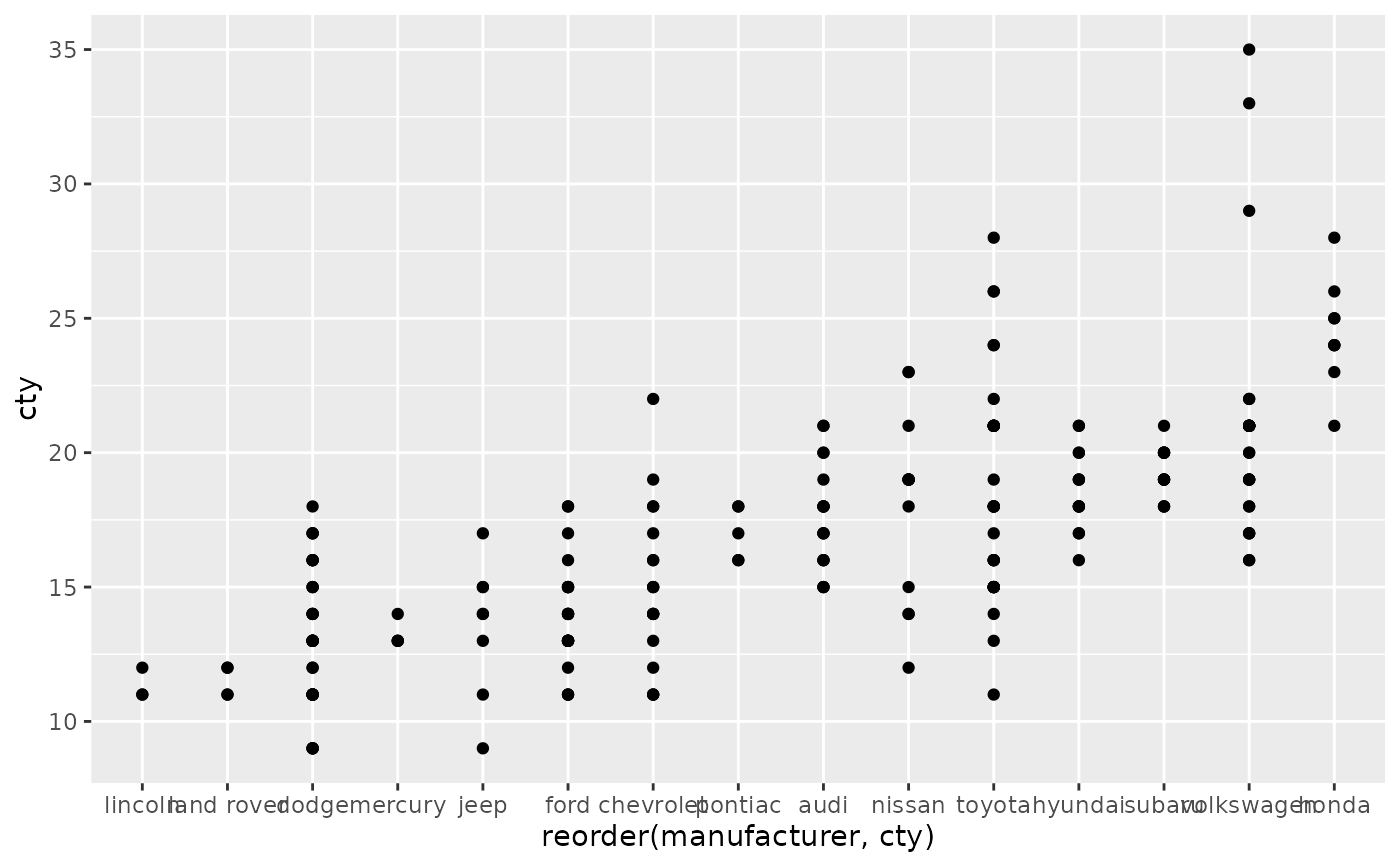ggplot(mpg, aes(reorder(manufacturer, displ), cty)) +
geom_point()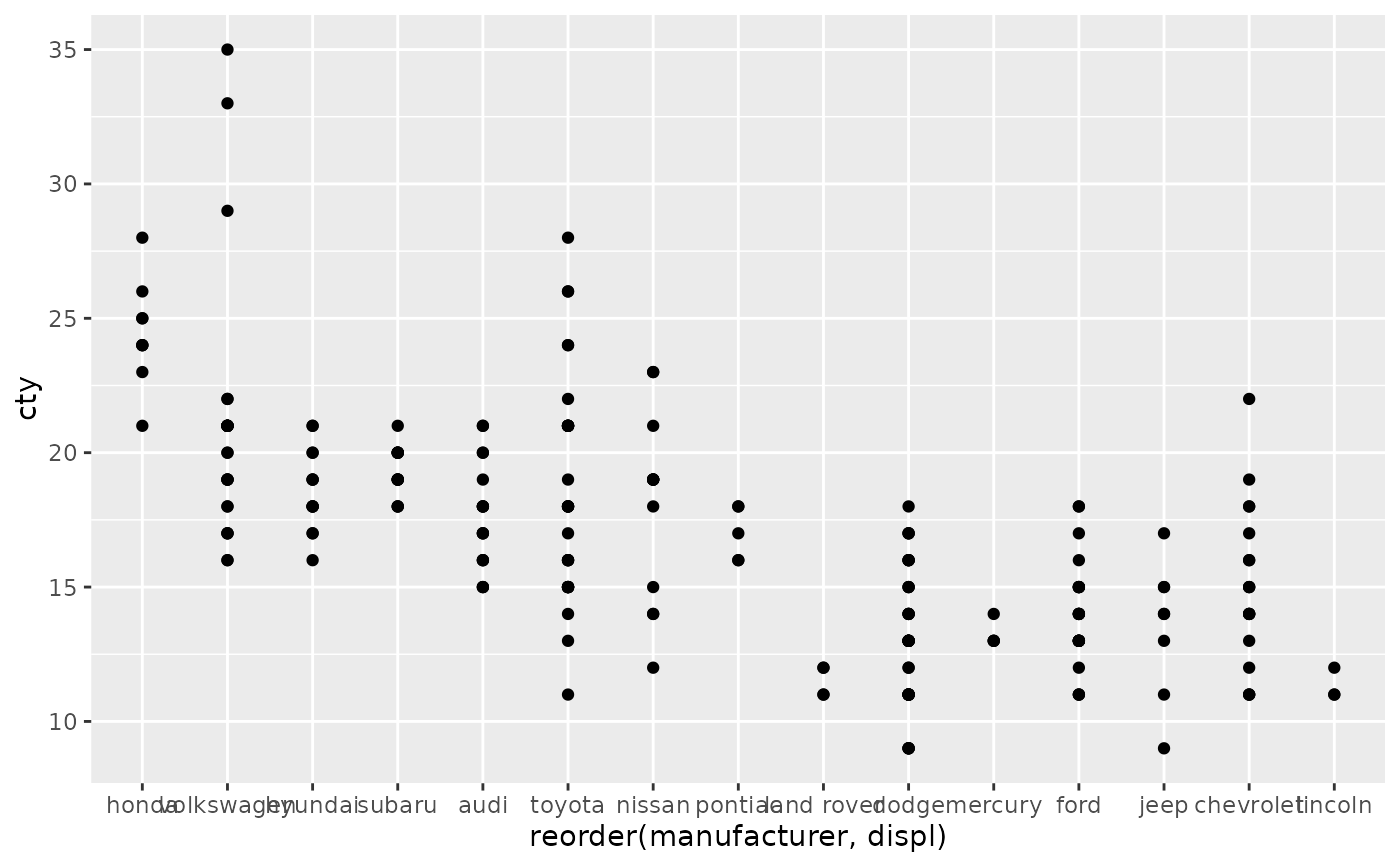# Use abbreviate as a formatter to reduce long names
ggplot(mpg, aes(reorder(manufacturer, displ), cty)) +
geom_point() +
scale_x_discrete(labels = abbreviate)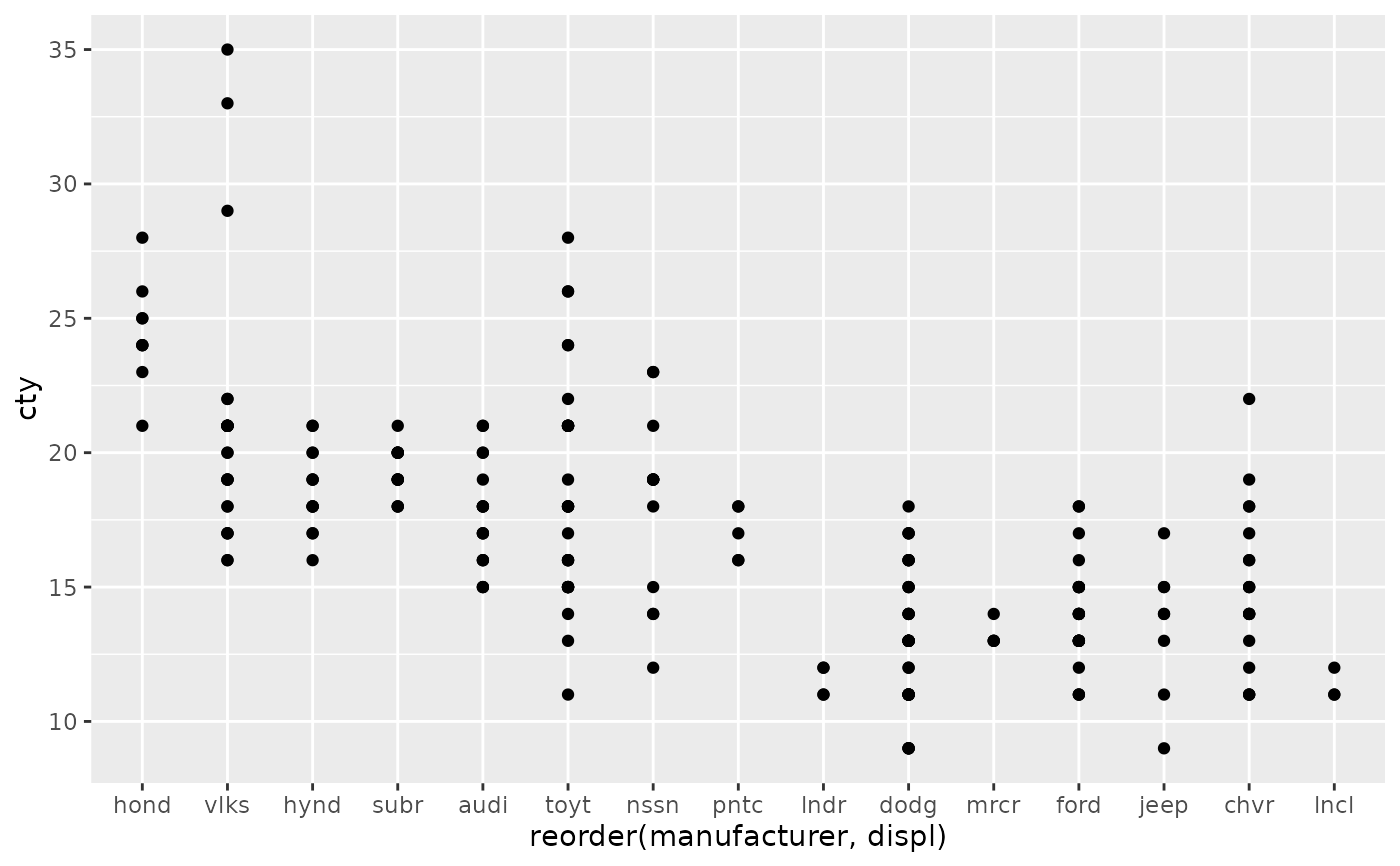# }